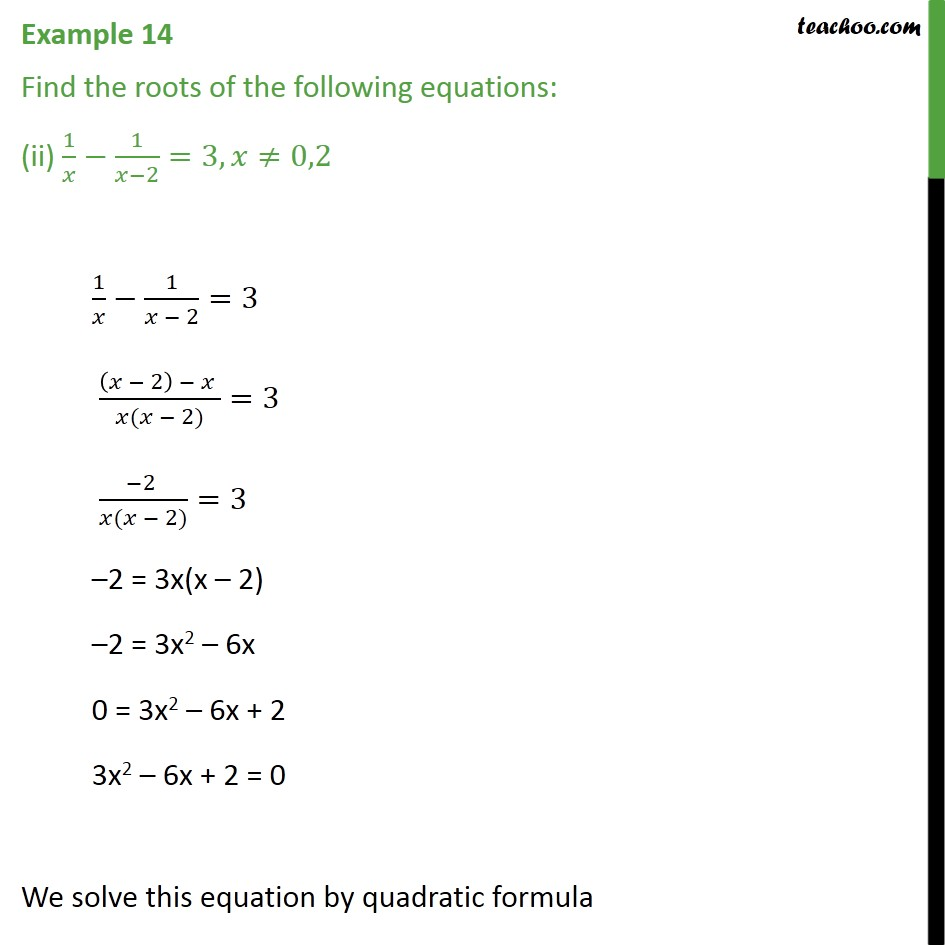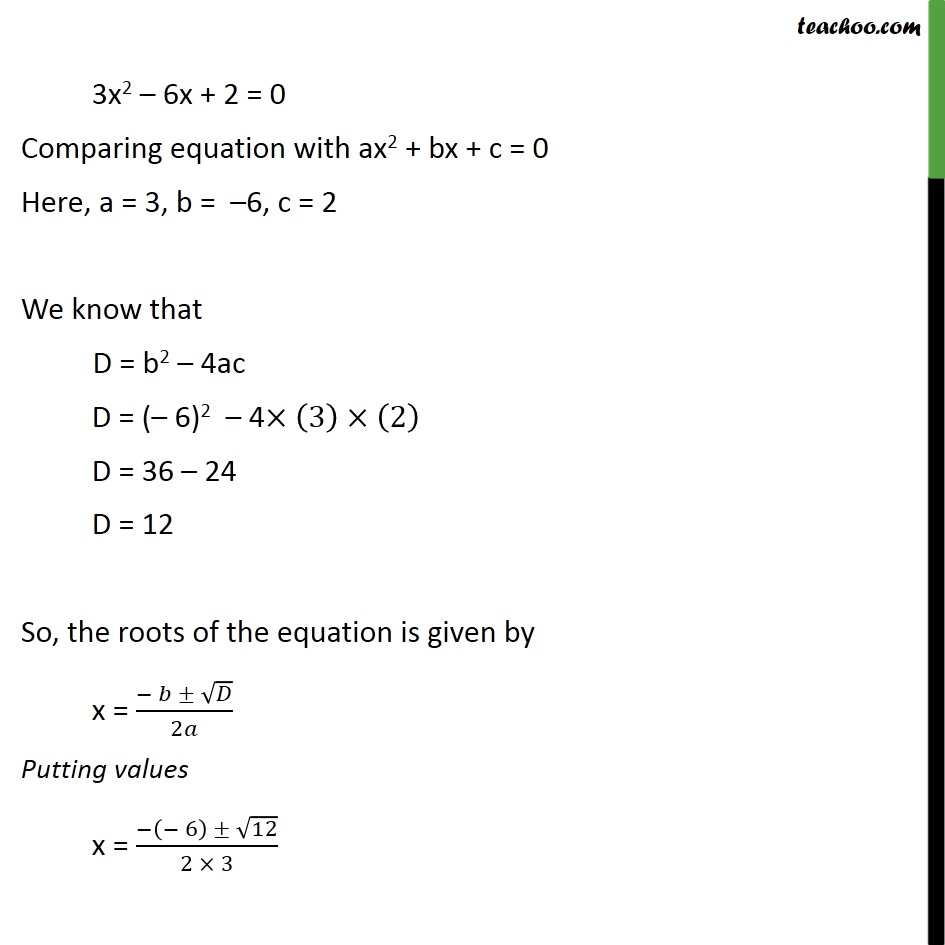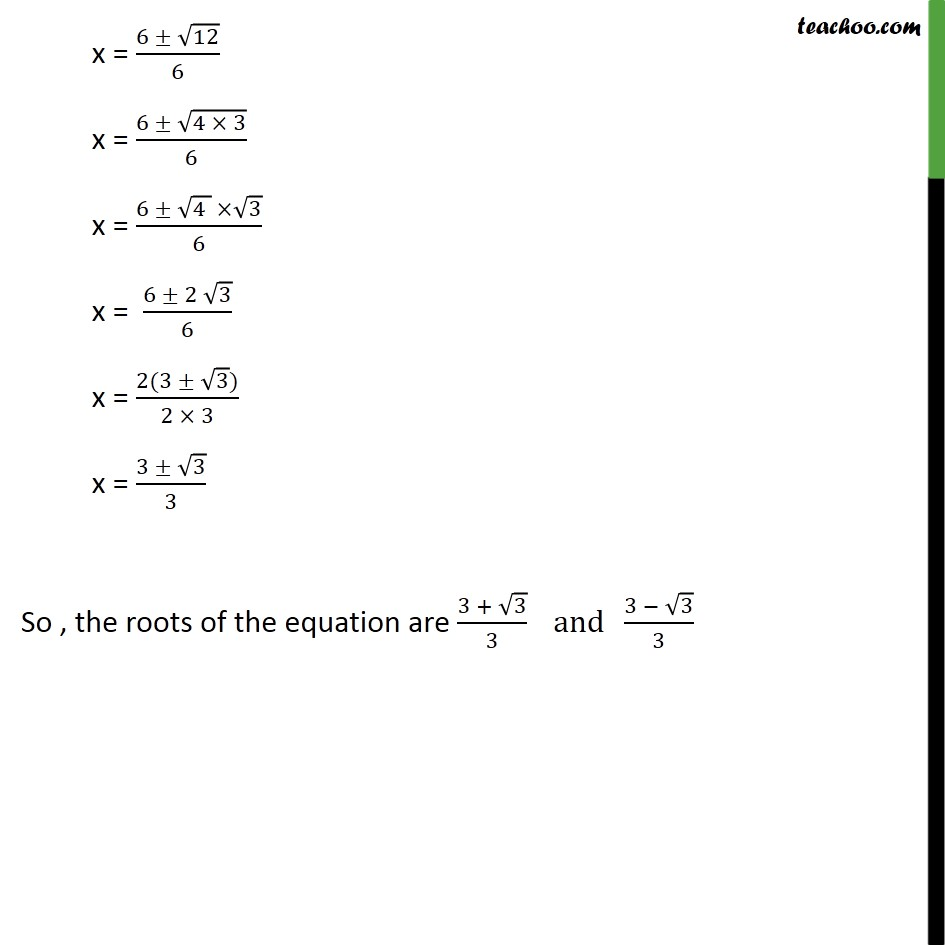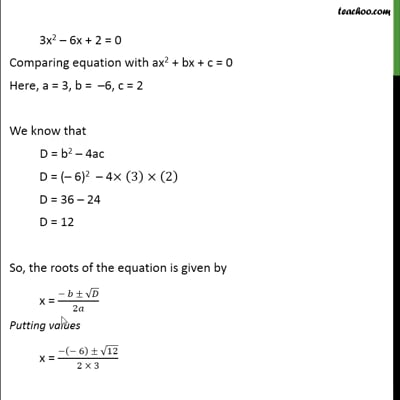Examples

Chapter 4 Class 10 Quadratic Equations
Serial order wiseThis video is only available for Teachoo black users

Maths Crash Course - Live lectures + all videos + Real time Doubt solving!

### Transcript

Example 14 Find the roots of the following equations: (ii) 1/𝑥−1/(𝑥−2)=3,𝑥≠0,2 1/𝑥−1/(𝑥 − 2)=3 ((𝑥 − 2) − 𝑥 )/(𝑥(𝑥 − 2))=3 (−2 )/(𝑥(𝑥 − 2))=3 –2 = 3x(x – 2) –2 = 3x2 – 6x 0 = 3x2 – 6x + 2 3x2 – 6x + 2 = 0 We solve this equation by quadratic formula 3x2 – 6x + 2 = 0 Comparing equation with ax2 + bx + c = 0 Here, a = 3, b = –6, c = 2 We know that D = b2 – 4ac D = (– 6)2 – 4×(3)×(2) D = 36 – 24 D = 12 So, the roots of the equation is given by x = (− 𝑏 ± √𝐷)/2𝑎 Putting values x = (−(− 6) ± √12)/(2 × 3) x = (6 ± √12)/6 x = (6 ± √(4 × 3))/6 x = (6 ± √(4 ) ×√3)/6 x = (6 ± 2 √3)/6 x = (2(3 ± √3))/(2 × 3) x = (3 ± √3)/3 So , the roots of the equation are (3 + √3)/3 and (3 − √3)/3